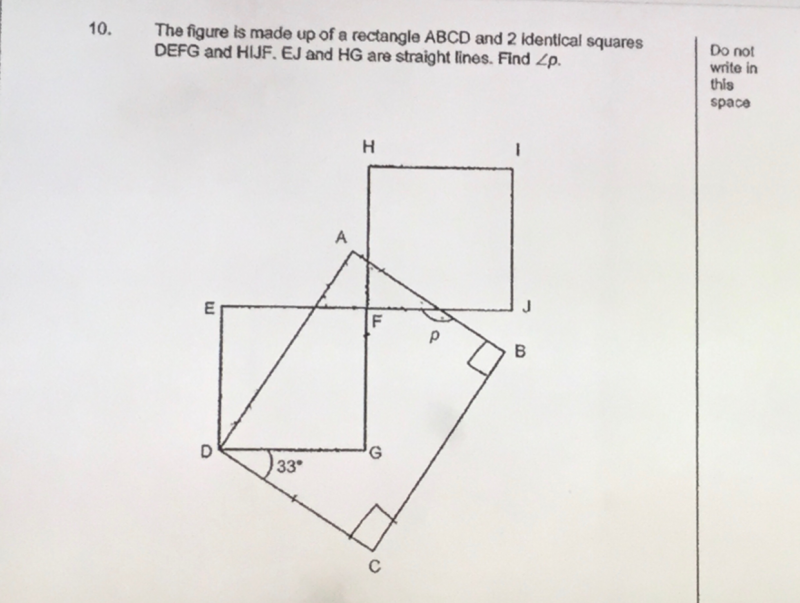# QuestionPls help to solve. Thanks!

Angle ADG = 90-33 = 57

Name the point between E and F, O

Then Angle AOF = Angle ADG = 57    (corresponding angles, EF//DG)

Therefore, angle p = 90+57 = 147o   (ext. angle = sum of opp 2 int. angles in a triangle)

0 Replies 2 Likes

147

0 Replies 0 Likes